Informatiker Board » Themengebiete » Theoretische Informatik » N = Np » Antwort erstellen » Hallo Gast [Anmelden|Registrieren]

Antwort erstellen
Benutzername: (du bist nicht eingeloggt!)
Thema:
Nachricht:

 HTML ist nicht erlaubtBBCode ist erlaubtSmilies sind erlaubtBilder sind erlaubt

 Smilies: 21 von 33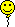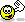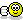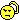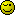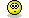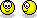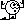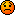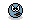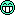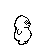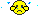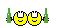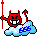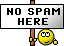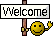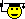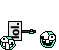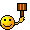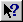aktuellen Tag schließen alle Tags schließen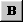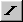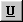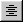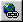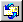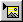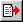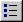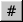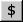Spamschutz:
Text aus Bild eingebenDer letzte Beitrag Anonycat90 N = NpMeine Frage: N = PN I discovered a wonderful algorithm and wanted to share it with you all. First we need to assume that string theory fully explains the phenomenon of W boson superheavy. We use the mass operator of string theory to correct the standard mass of the W boson. If the magnitude of the correction can eliminate 7 confidence intervals, it proves that the string theory is based on experimental facts. At this time, we need to review the algorithm of the Hamiltonian graph I wrote earlier, which was mentioned in my previous blog post on Facebook. We need to construct the topology of a simple "1+2+3" instance, which will be in the shape of a Möbius strip. First, we need to know that this structure is the simplest instance of a Hamiltonian graph. After that, we construct the conjecture of the 24 Umbral moonshine conjecture. It should be noted that the 24 Umbral moonshine conjecture here are all in line with Ramanujan's third-order simulation equation. If we use the Möbius strip of the "1+2+3" topology, we can easily slide a rule that a third-order equation must correspond to a first-order equation and a second-order equation. In this case, we can simplify it like this The third-order Ramanujan simulation equation, at this time, we can complete the simulation of 24 string effects through the mass operator of string theory. I think boson quantum computers are not the future, but the present. Meine Ideen: N = NP Anonycat90 hat dieses Bild (verkleinerte Version) angehängt: